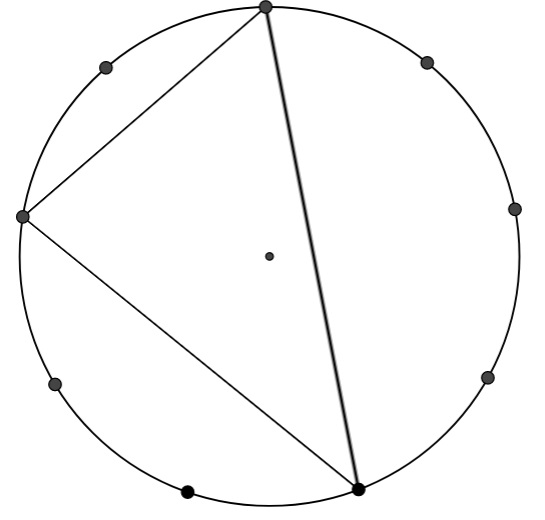#### You may also like### Coins on a Plate

Points A, B and C are the centres of three circles, each one of which touches the other two. Prove that the perimeter of the triangle ABC is equal to the diameter of the largest circle.### Not So Little X

Two circles are enclosed by a rectangle 12 units by x units. The distance between the centres of the two circles is x/3 units. How big is x?This gives a short summary of the properties and theorems of cyclic quadrilaterals and links to some practical examples to be found elsewhere on the site.

# Triangles in Circles

### Why do this problem ?

This problem challenges students to apply what they know about angles in triangles. The nine-point circle allows students to concentrate on the geometrical structure while keeping the arithmetic reasonably straightforward.

It offers a good preparation for the problems Subtended angles, Right angles, and Cyclic Quadrilaterals, which lead to proofs of circle theorems.

Teachers may find the article Angle Measurement: an Opportunity for Equity of interest.

### Possible approach

You can print out 9-point Circles for students to work on, or use the GeoGebra applet.

"How many different triangles can you make which consist of the centre point and two of the points on the edge? "
"Can you find all the angles of the triangles?"

At some stage students will want to know what makes triangles "different". This is a good opportunity to talk about congruence.

After a short time bring the class together to discuss how they calculated the angles.Next, draw a triangle with three vertices on the edge of the circle which encloses the centre point, such as the one illustrated here.

"Using what you already know, can you find all the angles of this triangle?"

After a few minutes, bring the class together to discuss what strategies they have used. It may be necessary to draw in the isosceles triangles to help students to relate this problem to what they have already found out.

Once they have a strategy, invite them to find as many different triangles as they can, and work out the angles. At some stage, you may need to discuss how to cope with triangles that do not enclose the centre.

### Key questions

What do you know that might be useful?
Are there any extra lines you can draw, to help make the problem more like something you've seen before?

### Possible extension

Subtended angles, Right angles, and Cyclic Quadrilaterals are good follow-up problems that lead on to proofs of circle theorems.

### Possible support

Some students may benefit from working on some of the Angles, Polygons and Geometrical Proof Short Problems in preparation for this task.